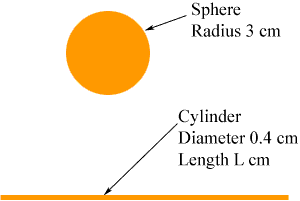Quandaries and Queries The radius of a copper sphere is 3cm. If  a wire of  0.4 diameter is drawn by melting it,what is the length of this wire? Hi, When you change the shape of the copper from a sphere to a long cylindrical wire, the amount of copper doesn't change. By the amount I mean the volume so the volume of the copper sphere and the volume of the cylinder are the same.The volume of the sphere is  4/3r3 =  4/333 = 36The volume of the cylinder isr2 h =0.042L Since the volume is unchanged 36=0.042L Solve for L. Penny Go to Math Central